### IMO Shortlist 1998 problem C4

Kvaliteta:
Avg: 0,0
Težina:
Avg: 7,0
Let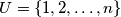$U=\{1,2,\ldots ,n\}$, where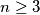$n\geq 3$. A subset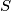$S$ of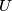$U$ is said to be split by an arrangement of the elements of$U$ if an element not in$S$ occurs in the arrangement somewhere between two elements of$S$. For example, 13542 splits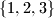$\{1,2,3\}$ but not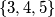$\{3,4,5\}$. Prove that for any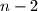$n-2$ subsets of$U$, each containing at least 2 and at most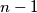$n-1$ elements, there is an arrangement of the elements of$U$ which splits all of them.
Izvor: Međunarodna matematička olimpijada, shortlist 1998